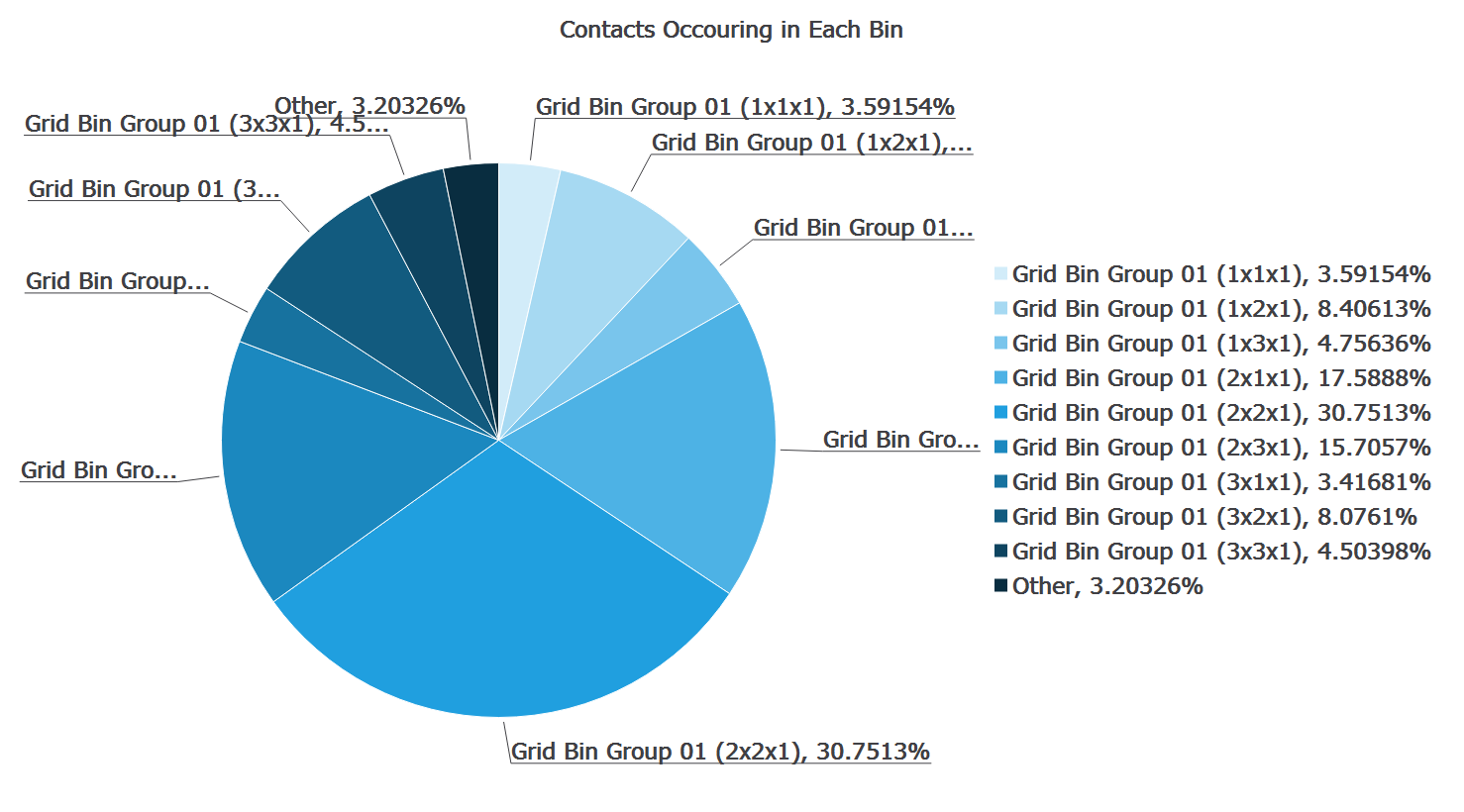# Creating a Pie Chart

A pie chart is a circular diagram divided into segments, each representing a category or subset of data (part of the whole). The amount for each category is proportional to the area of the sector. The total area of the circle is 100% and it represents the total population that is being shown.

For example, the figure below is a pie chart which shows the force on the equipment, broken down by equipment type, this is in a conveyor simulation and the chute experiences the greatest force, followed by the feed conveyor.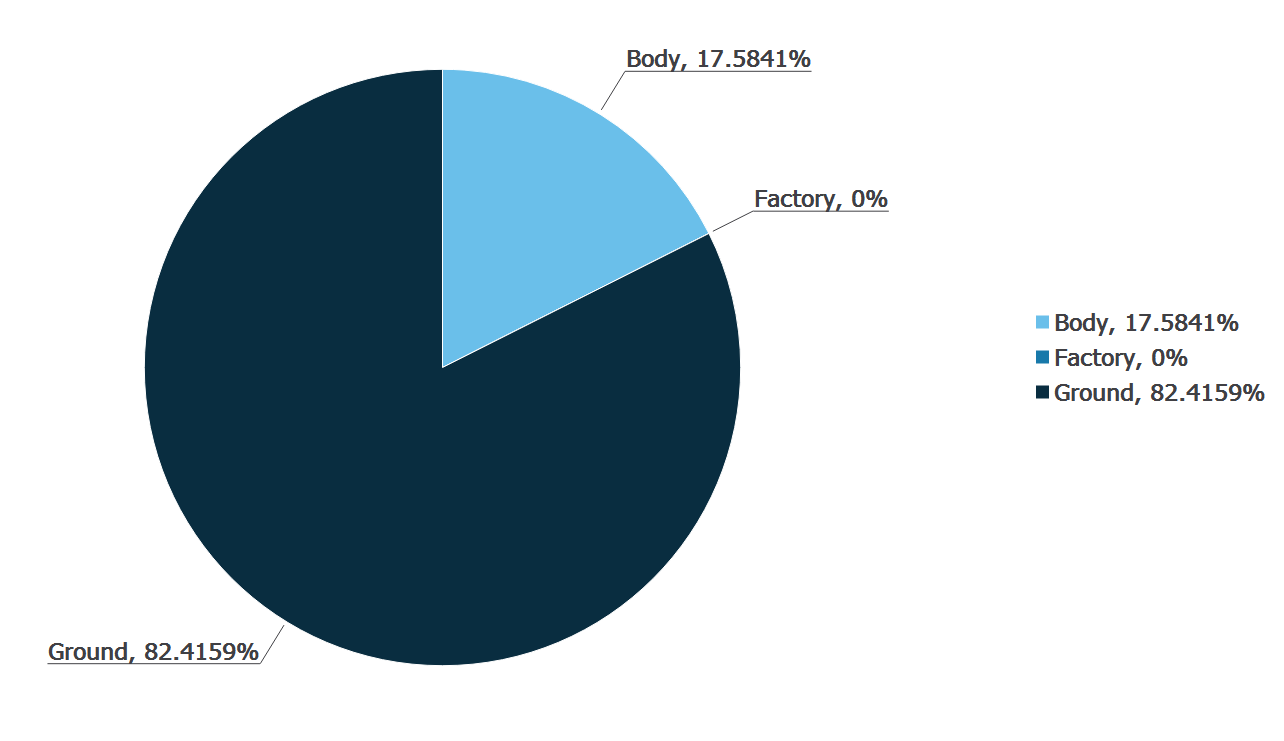## Selecting the Elements to Graph

The Select Element section is used to determine which elements in your model to collect data from.

Choose the group of elements you are interested in. For each element type, you can choose either to split the data by type or selection/bin group and these will form the segments (categories) of the pie chart.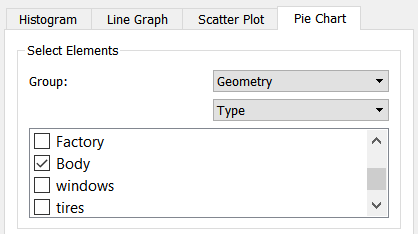When Type or Selection Group is selected, the categories within that selection are listed: For example if Contacts > Type are selected, all specific contacts are the categories listed: For example, particle x - particle x, particle x - particle y and particle y - particle y. Similarly if Contacts > Selection groups is selected, all selection and bin groups in your model are the categories listed. All categories within a type or group are included in the chart by default.

## Define Attribute

Click on the Select Attribute tab and select the element attribute and component you want to examine using the pie chart. The attributes available in the list will depend on the elements previously selected. The component type available for most attributes is Total as this is the only type that can be displayed using a pie chart: for example, the total mass of particles of Type A compared to particles of Type B. Refer to Appendix: Attribute Definitions for more information on attributes.

 Element Attribute Components Contacts Contact vector 1, 2. Magnitude, X, Y, Z. Normal force. Magnitude, X, Y, Z. Normal overlap. N/A Number of contacts. N/A Tangential force. Magnitude, X, Y, Z. Tangential overlap. N/A Custom property. Depends on number of elements. Collisions Average normal force. Magnitude, X, Y, Z. Average tangential force. Magnitude, X, Y, Z. Maximum normal force. Magnitude, X, Y, Z. Maximum tangential force. Magnitude, X, Y, Z. Normal energy loss. N/A Number of collisions. N/A Relative velocity. Magnitude, X, Y, Z. Relative velocity normal. Magnitude, X, Y, Z. Relative velocity tangential. Magnitude, X, Y, Z. Tangential energy loss. N/A Total energy loss. N/A Velocity of element A. Magnitude, X, Y, Z. Velocity of element B. Magnitude, X, Y, Z. Geometry Compressive force. N/A Distance N/A Pressure N/A Total force. Magnitude, X, Y, Z. Velocity Magnitude, X, Y, Z. Custom property. Depends on number of elements. Particle Angular velocity. Magnitude, X, Y, Z. Compressive force. N/A Diameter N/A Distance Define reference object*. Kinetic energy. N/A Mass N/A Number of particles. N/A Potential energy. N/A Rotational kinetic energy. N/A Torque Magnitude, X, Y, Z. Total energy. N/A Total force. Magnitude, X, Y, Z. Velocity Magnitude, X, Y, Z. Volume N/A Custom property. Depends on number of elements. Bond Normal force. Magnitude, X, Y, Z. Normal moment. Magnitude, X, Y, Z. Number of broken bonds. N/A Number of intact bonds. N/A Tangential force. Magnitude, X, Y, Z. Tangential moment. Magnitude, X, Y, Z.

* If the attribute is set to Distance you must define a point or plane from which the distance is measured. When distance is selected the Define Reference Object section of the pane will be activated. Choose the point or plane from which the distance should be measured. A point is defined by its xyz position and a plane by its orientation and distance from the origin.

## Settings

Two options are set in the Setting tab.

1. Time Step
Pie charts can be created for elements in a particular time step or over a range of time steps. By default the Current Time step option is selected. The current time step can be changed using the Current Time control in the Viewer control pane at the right of the screen.
For example, you could chart the total number of particles in each bin in a bin group at a particular time or the total number that have been in the bins over the course of the entire simulation. Comparing the charts we can see that at t=20s most particles are in bins (2x1x1) and (2x2x1); however, this is not indicative of their location over the course of the whole simulation.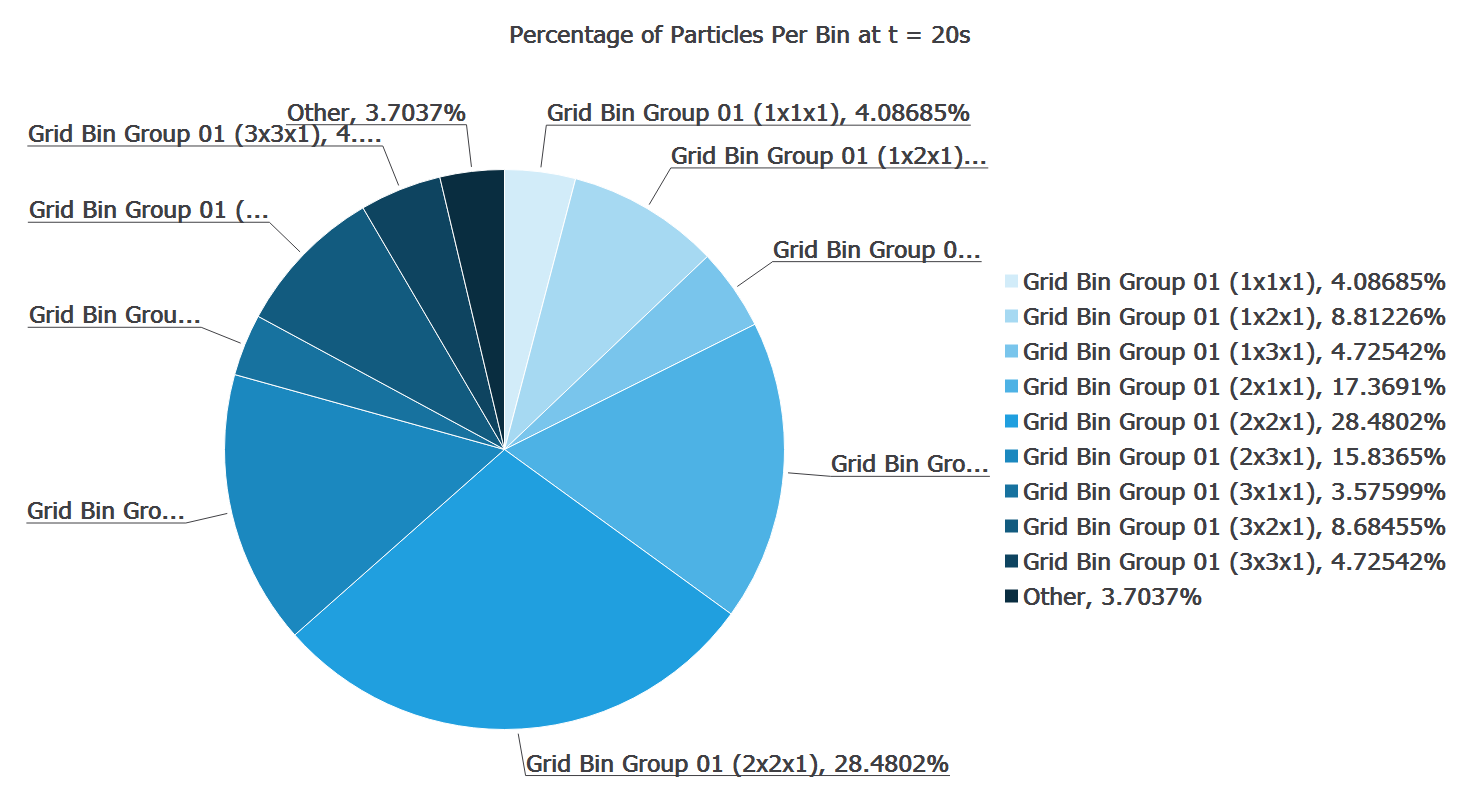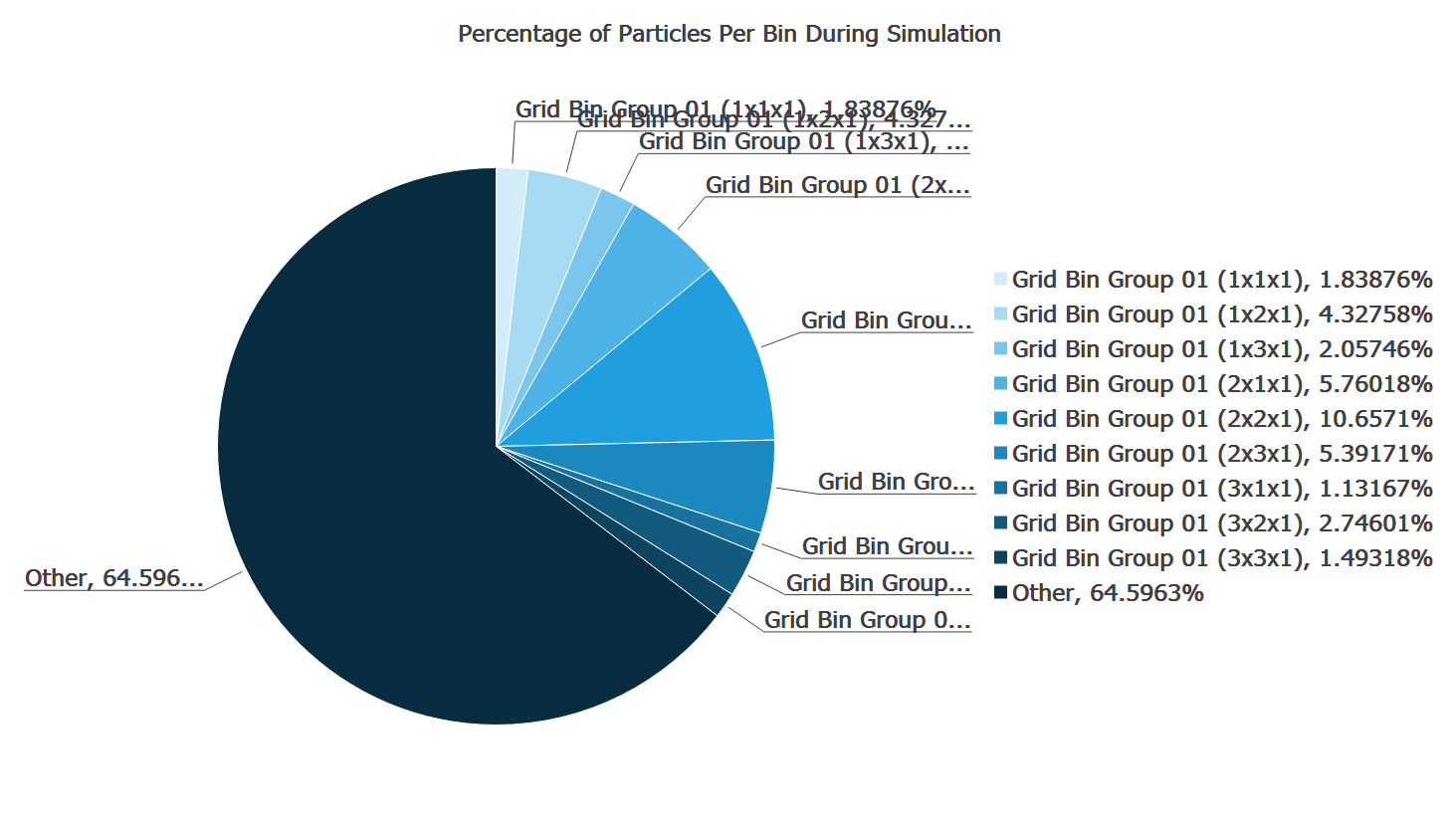Unselect the Current Time Step to set a different start and end time. Grayed-out time steps indicate partial saves and may not contain all the data you want to plot.

1. Graph Text
Set the pie chart title.

## Example Pie Chart of Bin Groups

A model contains a bin group dividing the model domain into 10 bins. You want to show the number of contacts that are occurring in each bin at a given time.

1. Select the element Contact > Selection.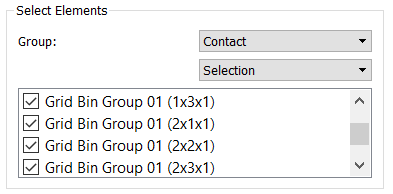2. Choose the attribute Number of Contacts (Total).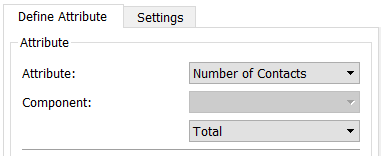3. Go to the Settings tab and give the chart a title.

4. Go to the Viewer Controls pane and choose the time step at which you want to examine the contacts. Ensure the Current time step option in the Settings tab is selected.
5. Click the Create Graph button.
6. The pie chart and the percentage breakdown of the number of contacts occurring in each bin will be displayed in the Viewer. For example: